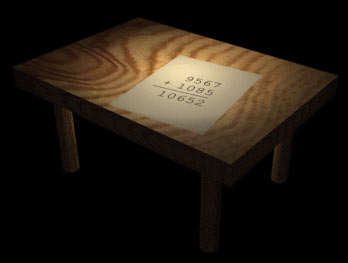# More Money`  SEND+ MORE------ MONEY`
This cryptogram is amusing in that it spells out a meaningful message and has a unique solution. Deciphering the solution takes some persistent logical reasoning, although inspired guesswork can speed up the process.

The obvious initial assumption is that M equals 1. From the fact that SEND #+ MORE is greater than 9,999 we can conclude that S is either 8 or 9, because S + 1 (or 2, if there was a carry from the previous column) is greater than 9. Knowing this yields an upper limit for O; it must be either 0 or 1. Since M is 1, O must be 0. The code now looks like this:

```  SEND
+ 10RE
------
10NEY
```
The same logic gives us a value for S: if S is 8, then E plus zero is greater than 10 and N is less than 2. (For S to be 8, there must have been a carry) However, N is not less than 2, because all lower values are accounted for. Therefore S is 9. Knowing that there was a carry tells us that E equals N - 1. Looking at the middle two columns we see that EN plus R, plus a possible carry, equals NE. R must therefore be equal 8 or 9, depending upon the carry. Seeing that 9 is taken, R must be 8:

Looking at the one's column (D and E), we know there was a carry from this column, so D plus E is greater than 11 (10 and 11 being ruled out because they end with 0 and 1). Furthermore, 8 and 9 are accounted for, so D and E could be at most 6 and 7 in either combination. We also can place a minimum value at 5 for either one, if the other is 7. We also know that E cannot be 7, since it is one less than N. This means D cannot be 5. So E can be either 5 or 6.

Assume that E is 6; D must be greater than 5, and less than 8. Six is taken by E (in our assumption) leaving D equal to 7. But if E is 6, N must be 7. So from the contradiction, we know is not 6, therefore it is 5.

This makes N equal to 6, D equal to 7 and Y equal to 2. The "message" translates to:

```  9567
+ 1085
------
10652
```

The student needed \$10,652. We know this because the method used to solve the problem eliminated the possibility of alternate solutions. So SEND + MORE = MONEY as a monoalphabetic cipher has a unique solution.

Updated 3/27/1999:

Some people have pointed out that there is an alternate solution if a number can begin with zero. True, but why waste the extra character? I believe (could be wrong) telegrams are by the character, not word.

3.42 stars. Votes are updated daily.

On a scale of 1 to 5, 1 being among your least favorite, 5 being among your most favorite, how would you rate this puzzle?

1 2 3 4 5